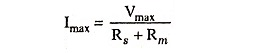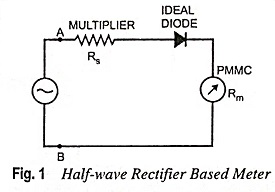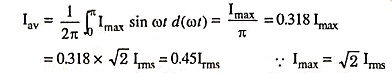## Electronic Instruments (Analog as well as Digital) Interview Questions and Answers:

1. Why ordinary voltmeter cannot be used in electronic circuits?

Ans. A moving coil voltmeter draws a large current, hence a large power from the test circuit during measurement. Generally current drawn by the voltmeter does not make much difference in electrical circuit but when measurement is involved in electronic circuits, current drawn by the voltmeter load the test circuit, and therefore, voltmeter shows an error in the reading,

2. Why is an electronic voltmeter more accurate than an ordinary voltmeter?

Ans. In electronic voltmeters power required for deflection of a permanent magnet moving coil (PMMC) movement is not drawn from the test circuit but supplied from the amplifier whose output is proportional to the test circuit voltage i.e. input to the amplifier, so power drawn from the test circuit becomes almost negligible. This feature makes the electronic voltmeter more accurate than an ordinary voltmeter.

3. Why are electronic instruments becoming more and more popular as compared to electrical instruments?

Ans. The electronic instruments are becoming more and more popular as compared to electrical instruments because of their inherent advantages such as high input impedance, extremely wide frequency range (10 Hz-100 MHz or even higher), high sensitivity, reduced loading effect, faster response, higher accuracy, flexibility. Electronic voltmeters are capable of measuring voltages of the order of microvolts as well as hundreds or thousands of volts and monitor remote signals.

4. What is full of VTVM?

Ans. VTVM stands for vacuum tube voltmeter.

5. List various types of TVMs?

Ans.

2. TVM with FET (field-effect transistor).
3. Balanced bridge TVM.
4. Chopper amplifier.

6. Where is VTVM used?

Ans. VTVMs are used for the measurement of the dc and ac voltages.

7. What is difference between TVM and VTVM?

Ans. Electronic voltmeter using vacuum tube is called a VTVM while that using a transistor is called a TVM.

8. In what respect TVM is inferior to VTVM?

Ans. The only disadvantage with TVM is its low input impedance which it offers in comparison to VTVM. But this shortcoming is overcome by using FET in input stage of voltmeter.

9. What are the advantages of TVM over VTVM?

Ans. The advantages of TVMs over VTVMs are given below:

1. A TVM does not require any warm up time because of absence of heating element.
2. Use of transistor makes the instrument portable because of its light weight.
3. A transistorized instrument can be operated on low voltage i.e. on battery so TVM is well suited for the fieldwork where power source is not available.
4. VTVM cannot measure current directly because of its high resistance whereas TVM can do.
5. TVM consumes very less power because of absence of heating element.

10. Differentiate between dc and ac voltmeters?

Ans. Fundamentally, dc and ac voltmeters are not much different, dc voltmeter consists of a dc amplifier succeeded by a conventional dc meter while an ac voltmeter consists of an additional rectifier either before or after the amplifier.

11. Why is direct-coupled amplifier dc voltmeter commonly used?

Ans. Because of its low cost.

12. What are the shortcomings of a direct-coupled dc voltmeter?

Ans. The drawback of a direct-coupled dc voltmeter is that it has to work under specified ambient temperature to have the required accuracy otherwise excessive drift problem occurs during operation. It can be used only for measuring voltage of the order of millivolts owing to limited amplifier gain. The drift problem in direct-coupled dc amplifiers restricts the use of a number of stages required for a high gain.

Ans. Main advantages associated with average reading ac voltmeters are that they are simple in construction, have high input impedance, low power consumption and uniform scale.

14. How can an average reading VTVM be converted to an RMS reading voltmeter?

Ans. The average reading voltmeter can be converted into an RMS reading voltmeter by calibrating its meter scale in terms of the rms value of a sinusoidal wave.

Ans. Main disadvantage of average reading voltmeters is that they operate in audio-frequency range. In radio-frequency range, distributed capacitance of the high resistance R introduces error in the readings. Another disadvantage of such a voltmeter is that due to non-linear volt-ampere characteristic for lower voltage the readings of the voltmeter at lower voltage are not correct.

16. Why is dc amplifier associated with diode rectifier in peak reading ac voltmeters provided with stabilising means?

Ans. The dc amplifier associated with diode rectifier should be provided with the stabilising means in order to prevent drift in the indication of the output meter.

17. For what type of measurement is true rms reading voltmeter required?

Ans. For measuring rms value of nonsinusoidal waveform a true rms reading voltmeter is required. It gives a meter indication by sensing heating power of waveform which is proportional to the square of the rms value of the voltage.

18. Why are FETs used in differential amplifier type of electronic voltmeter?

Ans. FETs are used to enhance the input resistance in differential amplifier type electronic voltmeter. They also isolates the low resistance voltmeters from the circuit under test so as to avoid errors owing to loading effects.

19. What is VOM?

Ans. The volt-ohm-milliammeter (VOM) is another name of multimeter. It is also called AVO meter.

20. What is a multimeter?

Ans. The instrument, which contains a function switch to connect the appropriate circuits to the d’Arsonval movement, is often called a multimeter or ampere-volt-ohm (AVO) meter or volt-ohm­-milliammeter (VOM).

21. What is the use of a multimeter?

Ans. Multimeter is used for measurement of current (dc as well as ac), voltage (dc as well as ac) and resistance. With the external source, high resistance (exceeding 1 MΩ), inductance and capacitance can be measured with this instrument.

22. List the shortcomings of a multimeter.

Ans. It may be noted that the ac sensitivity of a half-wave ac meter is only 45 per cent of the dc sensitivity, therefore, the loading effect is greater while measuring ac voltages than that while measuring corresponding dc voltages.

The rectifying action depends upon the frequency to some extent due to capacitance effect and rectifier instruments show lower readings. Instrument indications may be in error by as much as 0.5 % decrease for every 1 kHz rise in frequency.

23. Why ac sensitivity of a half wave ac meter is only 45 per cent of the dc sensitivity?

Ans. A circuit of a voltmeter using a half-wave rectifier is depicted in Fig. 1. A and B are the input terminals. Pure sinusoidal signal with peak value Vmax is applied at the input. Assuming the diode to be ideal, the peak value of current through the meter is given bywhere Rs is the resistance of multiplier and Rm is the resistance of PMMC.If the frequency of the input signal is very low, the deflection of the meter actually follows the variation in current. However, if the frequency is high enough, the inertia of the moving parts prevents the pointer from following the variations in the current. The deflection actually converges upon the value of the current variation, which is given by the equationThus, the current through the meter is 0.45Vrms/Rs+Rwhich produces a deflection that is 0.45 times that produced with dc of equal magnitude, V.

Hence the sensitivity of a half-wave rectifier instrument with ac is 0.45 times its sensitivity with dc.

24. Why is the sensitivity of the ac scale of a multimeter less than the dc section?

Ans. The commercial multimeter often uses the same scale markings for both its dc and ac voltage ranges. Since the dc component of a sine wave for half wave rectification is equal to 0.45 times the rms value, in order to obtain the same deflection on corresponding dc and ac voltage ranges, the multiplier for the ac range must be lowered proportionately. That is why the sensitivity of the ac scale of a multimeter is less than the dc section.

25. Where is LCR meter used?

Ans. LCR meters are used to measure the values of inductance (L), capacitance (C) and resistance (R) directly.

Ans. Digital instruments are preferred over analog instruments because of their inherent advantages over analog instruments such as greater speed, increased accuracy, better resolution, reduction in operator errors, and ability to provide automatic measurements in system applications.

27. What is digital instrument?

Ans. A digital instrument indicates the measured value in the form of decimal digits.

28. What is the important equipment in digital instruments?

Ans. Important equipment used in digital instruments are A/D converter at the input and D/A converter at the output.

29. What is a digital voltmeter (DVM)?

Ans. A digital voltmeter displays the value of ac or dc voltages being measured directly as discrete numerals in the decimal number system.

30. What are the advantages of a digital voltmeter (DVM) ?

Ans. A digital voltmeter displays the value of ac or dc voltage in decimal number system thus eliminating the errors due to parallax and observational errors. The use of DVMs increases the speed with which the readings can be taken. Also the output of DVMs can be fed to memory devices for future purpose which is not available in electronic voltmeter. On account of development of IC technology, it has been possible to reduce the size, cost of DVM. In fact DVM is less costly than analog type.

31. Why is DVM considered to be accurate?

Ans. DVM is considered to be accurate because a DVM displays the value of ac and dc voltage under measurement directly as discrete numerals in the decimal number system. Numerical readout of DVMs is advantageous since it eliminates observational errors committed by operators. The errors on account of parallax and approximations are entirely eliminated. The use of DVMs increases the speed with which readings can be taken,

32. What is the principle of operation of a DVM?

Ans. A DVM comprises of a clock generator, a circuit that converts the voltage to be measured into pulses and a digital frequency counter.

33. Name the different types of DVMs.

Ans. Digital voltmeters may be (i) Ramp type DVM (ii) Integrating type DVM (iii) Successive approximation type DVM (iv) Dual slope integrating type DVM.

34. Why is dual slope ADC preferred in a digital voltmeter?

Ans. Dual slope ADC is preferred in a DVM because it has low cost and high accuracy.

35. What is meant by resolution of a digital instrument?

Ans. Resolution of a digital instrument is defined as the number of digit positions or simply the number of digits used in a meter. For example a three-digit meter display on the digital meter for 0-1 V range will be able to indicate from 000 to 999 mV, with the smallest increment (resolution) of 1 mV.

36. What is meant by ‘sensitivity’ of a digital instrument ?

Ans. Sensitivity of a digital meter is the smallest change in input which the meter is able to detect. Thus, it is the full-scale value of the lowest range multiplied by the resolution of the meter.

It is given as S = (fs)min x R

where (fs)min is the lowest full-scale value of the digital meter and R is the resolution in decimal.

37. What is DMM?

Ans. DMM (digital multimeter) is basically a digital voltmeter and may be used for the measurement of voltage, current (dc or ac) and resistance.

Scroll to Top